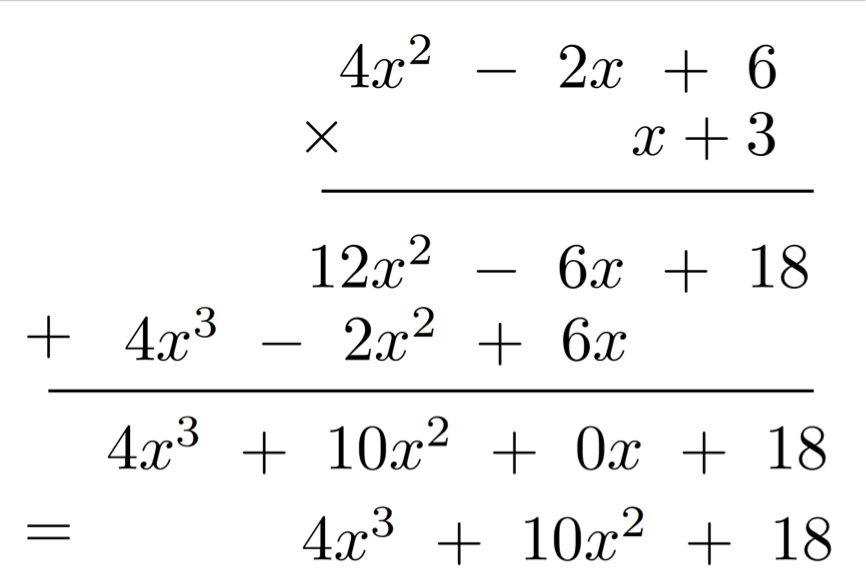# Alternative Strategies to Multiply Polynomials

Let’s try a couple of other methods that might make simplify the process for polynomials that are greater than two terms.

First, multiply the following numbers.

$$426\times 13$$

Write a list of steps that define the procedure you performed above.  Think about what you used to find the product (area model, distributive property, or traditional algorithm).

Did you do the following?

\begin{align} 426\\ \times \phantom{00}13 \\ \hline 1278 \\ 426\phantom{0} \\ \hline 5538\end{align}

Using the traditional algorithm, we can use the same procedures for multiplying polynomials .

We can use the same procedures for multiplying polynomials as shown below:

How is this strategy similar to the multiplication of the two digit numbers above?

How is this strategy like the distributive property?